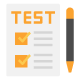Group QuestionAnswer the following question b...
Group Question
Answer the following question based on the information given below.
For a certain quiz, 10 questions have been short listed but the number of participating teams is yet undecided. The question numbers are labeled as Q1, Q2, Q3, Q4, Q5, Q6, Q7, Q8, Q9 and Q10.
Since the number of participating teams is not decided, it was decided to form sets from these 10 questions with the restriction that each set must have at least two questions.
However the selection of questions for any set was subject to following restrictions:
1. Q1 and Q8 must be in the same set.
2. Q8 and Q5 cannot be in the same set.
3. Q2 and Q6 cannot be in the same set.
4. Q9, Q2 and 0 4 if selected must be in the same set.
5. Q3 and Q10 must appear in the set together.
6. Q1 and Q3 cannot be in the same set.
Additional information for Q.1 and Q.2:
All the sets formed have 4 questions each.

Q. How many sets of four questions can be formed?
Group QuestionAnswer the following question based on the information g...
Possible sets having four questions each can be:
Set 1: Q3, Q10, Q5 and Q7
Set 2: Q9, Q2, Q4 and Q7
Set 3: Q6, Q5, Q3 and Q10
Set 4: Q6, Q7, Q3 and Q10
Set 5: Q9, Q2, Q4 and Q5
Set 6: Q1, Q8, Q6 and Q7
 1 Crore+ students have signed up on EduRev. Have you?
1 Crore+ students have signed up on EduRev. Have you?

### Learn this topic in detailCAT Practice Test - 27 100 Ques | 180 MinsMore from Related Course Quantitative Aptitude (Quant)View courses related to this question

### Quick links for CAT exam850+
Video Lectures2500+
Revision Notes600+
Online Tests10,000+
Doubts Solved
Group QuestionAnswer the following question based on the information given below.For a certain quiz, 10 questions have been short listed but the number of participating teams is yet undecided. The question numbers are labeled as Q1, Q2, Q3, Q4, Q5, Q6, Q7, Q8, Q9 and Q10.Since the number of participating teams is not decided, it was decided to form sets from these 10 questions with the restriction that each set must have at least two questions.However the selection of questions for any set was subject to following restrictions:1. Q1 and Q8 must be in the same set.2. Q8 and Q5 cannot be in the same set.3. Q2 and Q6 cannot be in the same set.4. Q9, Q2 and 0 4 if selected must be in the same set.5. Q3 and Q10 must appear in the set together.6. Q1 and Q3 cannot be in the same set.Additional information for Q.1 and Q.2:All the sets formed have 4 questions each.Q.How many sets of four questions can be formed?Correct answer is '6'. Can you explain this answer?
Question Description(Scan QR code)